# Sympy root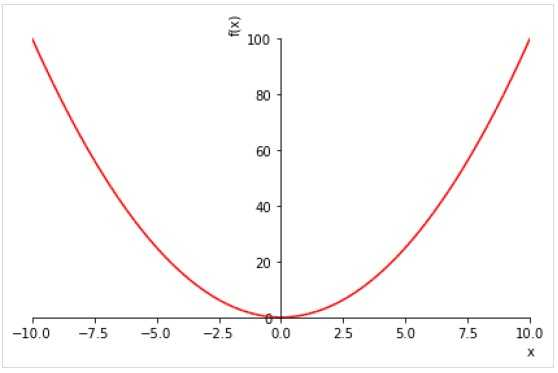Техника

This module implements elementary functions, as well as functions like ,
, etc.

## SymPy — Introduction

SymPy is a Python library for performing symbolic computation. It is a computer algebra system (CAS) that can be used either as a standalone application, as a library to other applications. Its live session is also available at https://live.sympy.org/. Since it is a pure Python library, it can be used as interactive mode and as a programmatic application. SymPy has now become a popular symbolic library for the scientific Python ecosystem.

Some of the areas of applications of SymPy are −

• Polynomials
• Calculus
• Discrete maths
• Matrices
• Geometry
• Plotting
• Physics
• Statistics
• Combinatorics

The roots function is for computing roots symbolically in radicals. It is usually not possible to compute roots in radicals for polynomials of degree 5 or more due to the Abel-Ruffini theorem. SymPy’s RootOf can represent those roots symbolically e.g.:

In : r = RootOf(x**25-96*x**12-4*x**3+2, 0)

In : r
Out:
⎛ 25       12      3       ⎞
CRootOf⎝x   - 96⋅x   - 4⋅x  + 2, 0⎠

In : r.evalf()
Out: -0.763733782729520


If you just want a numeric approximation of the roots then you can compute them all reasonable efficiently with the nroots method:

In : Poly(x**25-96*x**12-4*x**3+2, x).nroots()
Out:
[-0.76373378272952, 0.670586457724312, 1.42079389150078, -1.37945211815055 - 0.340175934440882⋅ⅈ, -1.37945211815055 + 0.34017593
4440882⋅ⅈ, -1.06319298080894 - 0.941998724697874⋅ⅈ, -1.06319298080894 + 0.941998724697874⋅ⅈ, -0.611146252548878 - 0.404661250727
9⋅ⅈ, -0.611146252548878 + 0.4046612507279⋅ⅈ, -0.503926321737819 - 1.32829285156355⋅ⅈ, -0.503926321737819 + 1.32829285156355⋅ⅈ, -
0.335293228608336 - 0.580740856215139⋅ⅈ, -0.335293228608336 + 0.580740856215139⋅ⅈ, -0.0448923539768514 - 0.73158885083763⋅ⅈ, -0.
0448923539768514 + 0.73158885083763⋅ⅈ, 0.171380585123656 - 1.41042748193864⋅ⅈ, 0.171380585123656 + 1.41042748193864⋅ⅈ, 0.3818668
72385803 - 0.66145201247479⋅ⅈ, 0.381866872385803 + 0.66145201247479⋅ⅈ, 0.656038617401695 - 0.326927596015531⋅ⅈ, 0.65603861740169
5 + 0.326927596015531⋅ⅈ, 0.807048139834163 - 1.16893577654226⋅ⅈ, 0.807048139834163 + 1.16893577654226⋅ⅈ, 1.25774575783827 - 0.66
0302613302175⋅ⅈ, 1.25774575783827 + 0.660302613302175⋅ⅈ]


The real_roots function supports finding the real roots of an univariate polynomial with rational coefficients e.g.:

In : real_roots(x**2 - 1)
Out: [-1, 1]

In : real_roots(x**2 + 1)
Out: []


Internally this is done in several stages:

1. The polynomial can be completely factorised over the rationals into irreducibles.
2. For each irreducible expressions for the roots are computed as either rational, radical or CRootOf.
3. Root isolation algorithms are used to identify which of those roots is real.

Note that step 1 immediately identifies all rational roots since they are reduced to linear factors. In step 2 the cubic and quartic formulas are not used but the quadratic formula is and decomposition and other techniques are possibly tried (not sure). You can use the roots function for the cubic and quartic formulas but they usually produce excessively complicated output. Anything not handled becomes CRootOf.

In step 3 the implementation can work for CRootOf meaning that it can also work for quintics and higher degree polynomials (it is not limited by Abel-Ruffini):

In : [r] = real_roots(x**5 - x - 1)

In : r
Out:
⎛ 5           ⎞
CRootOf⎝x  - x - 1, 0⎠

In : r.evalf()
Out: 1.16730397826142


The root isolation code is here:
https://github.com/sympy/sympy/blob/01a5776b9feaf01d06c94165ccebe4ac4b617a7b/sympy/polys/rootisolation.py

That code implements some fairly complex algorithms that are apparently quite fast but the implementation in sympy is very slow for higher degree polynomials. I am not sure if those algorithms are optimal for something like univariate polynomials or whether the implementation is just not well optimised. I think that an implementation based on the interval-Newton method could be much faster:

Symbolic computation refers to development of algorithms for manipulating mathematical expressions and other mathematical objects. Symbolic computation integrates mathematics with computer science to solve mathematical expressions using mathematical symbols. A Computer Algebra System (CAS) such as SymPy evaluates algebraic expressions exactly (not approximately) using the same symbols that are used in traditional manual method. For example, we calculate square root of a number using Python’s math module as given below −

>>> import math
>>> print (math.sqrt(25), math.sqrt(7))


As you can see, square root of 7 is calculated approximately. But in SymPy square roots of numbers that are not perfect squares are left unevaluated by default as given below −

>>> import sympy
>>> print (sympy.sqrt(7))


It is possible to simplify and show result of expression symbolically with the code snippet below −

>>> import math
>>> print (math.sqrt(12))


You need to use the below code snippet to execute the same using sympy −

##sympy output
>>> print (sympy.sqrt(12))


SymPy code, when run in Jupyter notebook, makes use of MathJax library to render mathematical symbols in LatEx form. It is shown in the below code snippet −

>>> from sympy import *
>>> x=Symbol ('x')
>>> expr = integrate(x**x, x)
>>> expr

Integral(x**x, x)


Which is equivalent to

>>> from sympy import *
>>> x=7
>>> sqrt(x)


A symbolic computation system such as SymPy does all sorts of computations (such as derivatives, integrals, and limits, solve equations, work with matrices) symbolically. SymPy package has different modules that support plotting, printing (like LATEX), physics, statistics, combinatorics, number theory, geometry, logic, etc.

## ITE function

This function acts as If then else clause in a programming language.ITE(A, B, C) evaluates and returns the result of B if A is true else it returns the result of C. All args must be Booleans.

>>> from sympy import * >>> from sympy.logic.boolalg import ITE >>> a,b,c=symbols('a b c') >>> a,b,c=(True, False, True)
>>> ITE(a,b,c), ITE(a,c,b)


## ExprCondPair¶

class sympy.functions.elementary.piecewise.ExprCondPair

Represents an expression, condition pair.

cond

Returns the condition of this pair.

expr

Returns the expression of this pair.

free_symbols

Return the free symbols of this pair.

## Inverse()

This method returns inverse of a quaternion object.

>>> q1.inverse()


The above code snippet gives an output equivalent to the below expression −

## SymPy — Derivative

The derivative of a function is its instantaneous rate of change with respect to one of its variables. This is equivalent to finding the slope of the tangent line to the function at a point.we can find the differentiation of mathematical expressions in the form of variables by using diff() function in SymPy package.

diff(expr, variable)
>>> from sympy import diff, sin, exp
>>> from sympy.abc import x,y
>>> expr=x*sin(x*x)+1 >>> expr


The above code snippet gives an output equivalent to the below expression −

$x\sin(x^2) + 1$

>>> diff(expr,x)


The above code snippet gives an output equivalent to the below expression −

$2x^2\cos(x^2) + \sin(x^2)$

>>> diff(exp(x**2),x)


The above code snippet gives an output equivalent to the below expression −

To take multiple derivatives, pass the variable as many times as you wish to differentiate, or pass a number after the variable.

>>> diff(x**4,x,3)


The above code snippet gives an output equivalent to the below expression −

>>> for i in range(1,4): print (diff(x**4,x,i))


The above code snippet gives the below expression −

It is also possible to call diff() method of an expression. It works similarly as diff() function.

>>> expr=x*sin(x*x)+1
>>> expr.diff(x)


The above code snippet gives an output equivalent to the below expression −

$2x^2\cos(x^2) + \sin(x^2)$

An unevaluated derivative is created by using the Derivative class. It has the same syntax as diff() function. To evaluate an unevaluated derivative, use the doit method.

>>> from sympy import Derivative
>>> d=Derivative(expr)
>>> d


The above code snippet gives an output equivalent to the below expression −

>>> d.doit()


The above code snippet gives an output equivalent to the below expression −

$2x^2\cos(x^2) + \sin(x^2)$

## IdentityFunction¶

class sympy.functions.elementary.miscellaneous.IdentityFunction

The identity function





## Basic manipulation

The shape property of Matrix object returns its size.

>>> M.shape


The row() and col() method respectively returns row or column of specified number.

>>> M.row(0)
$\displaystyle \left[\begin{matrix}10 & 40 & 30\end{matrix}\right]$

>>> M.col(1)
$\displaystyle \left[\begin{matrix}40\\6\end{matrix}\right]$


Use Python’s slice operator to fetch one or more items belonging to row or column.

>>> M.row(1)[1:3]
[6, 9]


Matrix class has row_del() and col_del() methods that deletes specified row/column from given matrix −

>>> M=Matrix(2,3,[10,40,30,2,6,9])
>>> M.col_del(1)
>>> M

Matrix([[10, 30],[ 2, 9]])

$\displaystyle \left[\begin{matrix}10 & 30\\2 & 9\end{matrix}\right]$

>>> M.row_del(0)
>>> M

$\displaystyle \left[\begin{matrix}2 & 9\end{matrix}\right]$


Similarly, row_insert() and col_insert() methods add rows or columns at specified row or column index

>>> M1=Matrix([[10,30]])
>>> M=M.row_insert(0,M1)
>>> M

$\displaystyle \left[\begin{matrix}10 & 30\\2 & 9\end{matrix}\right]$

>>> M2=Matrix([40,6])
>>> M=M.col_insert(1,M2)
>>> M

$\displaystyle \left[\begin{matrix}10 & 40 & 30\\2 & 6 & 9\end{matrix}\right]$


## Triangle

This function builds a triangle entity from three point objects.

>>> t=Triangle(Point(0,0),Point(0,5), Point(5,0))
>>> t.area


## SymPy — Integration

The SymPy package contains integrals module. It implements methods to calculate definite and indefinite integrals of expressions. The integrate() method is used to compute both definite and indefinite integrals. To compute an indefinite or primitive integral, just pass the variable after the expression.

For example −

integrate(f, x)

(integration_variable, lower_limit, upper_limit)

>>> from sympy import *
>>> x,y = symbols('x y')
>>> expr=x**2 + x + 1
>>> integrate(expr, x)


The above code snippet gives an output equivalent to the below expression −

>>> expr=sin(x)*tan(x)
>>> expr
>>> integrate(expr,x)


The above code snippet gives an output equivalent to the below expression −

The example of definite integral is given below −

>>> expr=exp(-x**2)
>>> integrate(expr,(x,0,oo) )


The above code snippet gives an output equivalent to the below expression −

You can pass multiple limit tuples to perform a multiple integral. An example is given below −

>>> expr=exp(-x**2 - y**2)
>>> integrate(expr,(x,0,oo),(y,0,oo))


The above code snippet gives an output equivalent to the below expression −

You can create unevaluated integral using Integral object, which can be evaluated by calling doit() method.

>>> expr = Integral(log(x)**2, x)
>>> expr


The above code snippet gives an output equivalent to the below expression −

>>> expr.doit()


The above code snippet gives an output equivalent to the below expression −

$x\log(x)^2 — 2xlog(x) + 2x$

## And function

A logical AND function evaluates its two arguments and returns False if either of them is False. The function emulates & operator.

>>> from sympy import *
>>> from sympy.logic.boolalg import And
>>> x,y=symbols('x y')
>>> x=True
>>> y=True
>>> And(x,y), x"&"y


### (True, True)

>>> y=False
>>> And(x,y), x"&"y


## SymPy — Lambdify() function

The lambdify function translates SymPy expressions into Python functions. If an expression is to be evaluated over a large range of values, the evalf() function is not efficient. lambdify acts like a lambda function, except it converts the SymPy names to the names of the given numerical library, usually NumPy. By default, lambdify on implementations in the math standard library.

>>> expr=1/sin(x)
>>> f=lambdify(x, expr)
>>> f(3.14)

>>> expr=a**2+b**2
>>> f=lambdify([a,b],expr)
>>> f(2,3)


However, to leverage numpy library as numerical backend, we have to define the same as an argument for lambdify() function.

>>> f=lambdify([a,b],expr, "numpy")


We use two numpy arrays for two arguments a and b in the above function. The execution time is considerably fast in case of numpy arrays.

>>> import numpy
>>> l1=numpy.arange(1,6)
>>> l2=numpy.arange(6,11)
>>> f(l1,l2)


## Miscellaneous Functions

Min − Returns minimum value of the list. It is named Min to avoid conflicts with the built-in function min.

Max − Returns maximum value of the list. It is named Max to avoid conflicts with the built-in function max.

root − Returns nth root of x.

sqrt − Returns the principal square root of x.

cbrt − This function computes the principal cube root of x, (shortcut for x++Rational(1,3)).

>>> Min(pi,E)

>>> Max(5, Rational(11,2))

>>> root(7,Rational(1,2))

>>> sqrt(2)

>>> cbrt(1000)


## SymPy — Plotting

pip install matplotlib

• plot − 2D line plots

• plot3d − 3D line plots

• plot_parametric − 2D parametric plots

• plot3d_parametric − 3D parametric plots

The plot() function returns an instance of Plot class. A plot figure may have one or more SymPy expressions. Although it is capable of using Matplotlib as backend, other backends such as texplot, pyglet or Google charts API may also be used.

plot(expr, range, kwargs)


where expr is any valid symPy expression. If not mentioned, range uses default as (-10, 10).

>>> from sympy.plotting import plot
>>> from sympy import *
>>> x=Symbol('x')
>>> plot(x**2, line_color='red')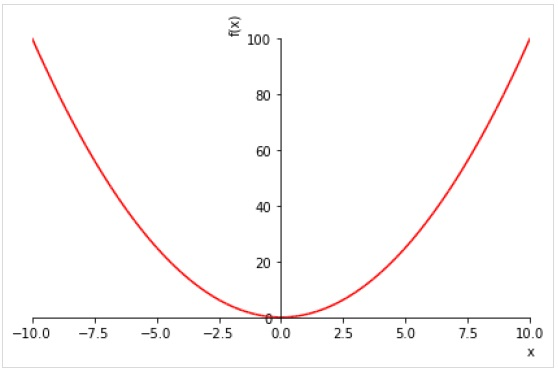To draw multiple plots for same range, give multiple expressions prior to the range tuple.

>>> plot( sin(x),cos(x), (x, -pi, pi))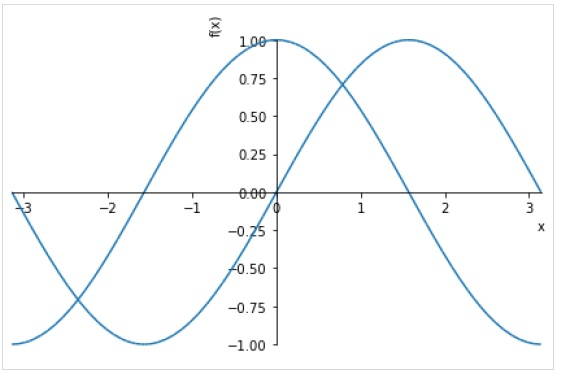You can also specify separate range for each expression.

plot((expr1, range1), (expr2, range2))

>>> plot( (sin(x),(x, -2*pi, 2*pi)),(cos(x), (x, -pi, pi)))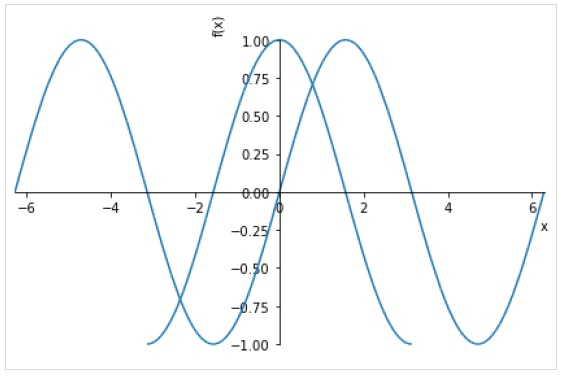• line_color − specifies color of the plot line.

• title − a string to be displayed as title

• xlabel − a string to be displayed as label for X axis

• ylabel − a string to be displayed as label for y axis

>>> plot( (sin(x),(x, -2*pi, 2*pi)),(cos(x), (x, -pi, pi)), line_color='red', title='SymPy plot example')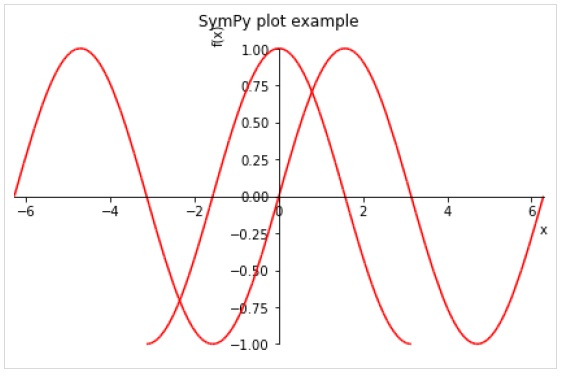The plot3d() function renders a three dimensional plot.

plot3d(expr, xrange, yrange, kwargs)

>>> from sympy.plotting import plot3d
>>> x,y=symbols('x y')
>>> plot3d(x*y, (x, -10,10), (y, -10,10))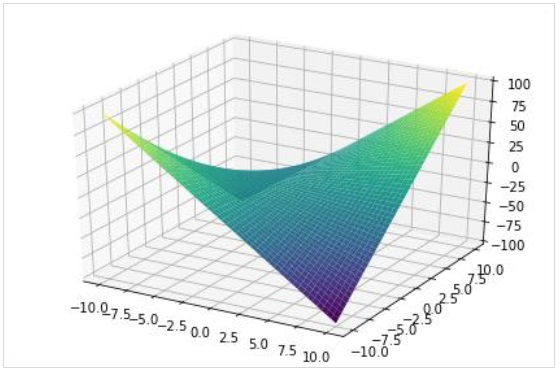As in 2D plot, a three dimensional plot can also have multiple plots each with different range.

>>> plot3d(x*y, x/y, (x, -5, 5), (y, -5, 5))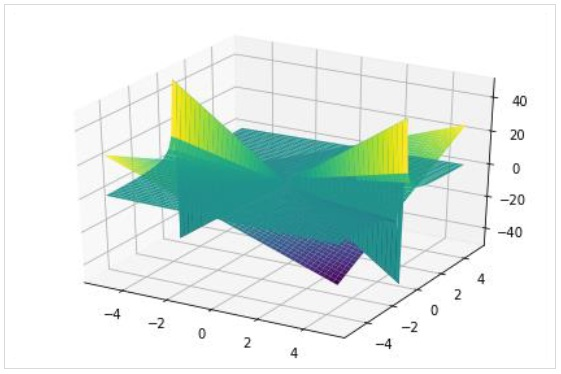The plot3d_parametric_line() function renders a 3 dimensional parametric line plot.

>>> from sympy.plotting import plot3d_parametric_line
>>> plot3d_parametric_line(cos(x), sin(x), x, (x, -5, 5))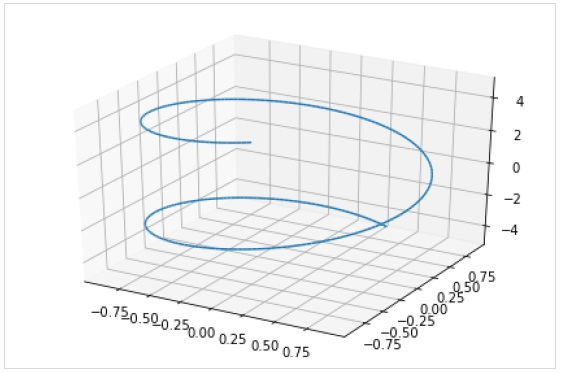To draw a parametric surface plot, use plot3d_parametric_surface() function.

plot3d_parametric_surface(xexpr, yexpr, zexpr, rangex, rangey, kwargs)

>>> from sympy.plotting import plot3d_parametric_surface
>>> plot3d_parametric_surface(cos(x+y), sin(x-y), x-y, (x, -5, 5), (y, -5, 5))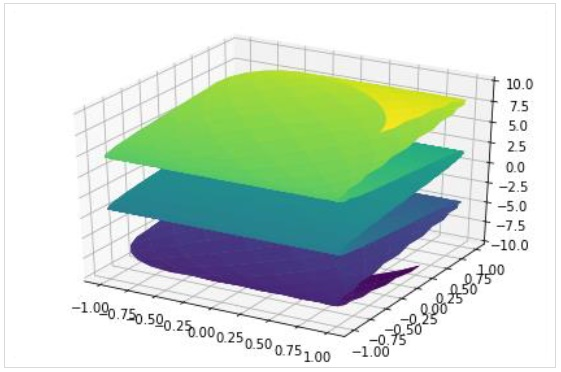## Abs¶

Returns the absolute value of the argument.




class sympy.functions.elementary.complexes.Abs

Return the absolute value of the argument.

This is an extension of the built-in function abs() to accept symbolic
values. If you pass a SymPy expression to the built-in abs(), it will
pass it automatically to Abs().



# The Python built-in



Note that the Python built-in will return either an Expr or int depending on
the argument:





Abs will always return a sympy object.

fdiff(argindex=1)

Get the first derivative of the argument to Abs().





## Or function

>>> from sympy import *
>>> from sympy.logic.boolalg import Or
>>> x,y=symbols('x y')
>>> x=True
>>> y=False
>>> Or(x,y), x|y


### (True, True)

>>> x=False
>>> y=False
>>> Or(x,y), x|y


## SymPy — Simplification

Sympy has powerful ability to simplify mathematical expressions. There are many functions in SymPy to perform various kinds of simplification. A general function called simplify() is there that attempts to arrive at the simplest form of an expression.

Дополнительно:  Ошибка синий экран Windows 10 – устранение проблемы /

## SymPy — Solvers

Since the symbols = and == are defined as assignment and equality operators in Python, they cannot be used to formulate symbolic equations. SymPy provides Eq() function to set up an equation.

>>> from sympy import *
>>> x,y=symbols('x y')
>>> Eq(x,y)


The above code snippet gives an output equivalent to the below expression −

Since x=y is possible if and only if x-y=0, above equation can be written as −

>>> Eq(x-y,0)


The above code snippet gives an output equivalent to the below expression −

x − y = 0

solveset(equation, variable, domain)

>>> solveset(Eq(x**2-9,0), x)

>>> solveset(Eq(x**2-3*x, -2),x)


The output of solveset is a FiniteSet of the solutions. If there are no solutions, an EmptySet is returned

>>> solveset(exp(x),x)


## RoundFunction¶

class sympy.functions.elementary.integers.RoundFunction

The base class for rounding functions.

## Functions on Integer Number

This is a set of functions to perform various operations on integer number.

This is a univariate function which returns the smallest integer value not less than its argument. In case of complex numbers, ceiling of the real and imaginary parts separately.

>>> ceiling(pi), ceiling(Rational(20,3)), ceiling(2.6+3.3*I)


The output for the above code snippet is given below −

(4, 7, 3 + 4*I)

This function returns the largest integer value not greater than its argument. In case of complex numbers, this function too takes the floor of the real and imaginary parts separately.

>>> floor(pi), floor(Rational(100,6)), floor(6.3-5.9*I)


The output for the above code snippet is given below −

(3, 16, 6 — 6*I)

This function represents the fractional part of x.

>>> frac(3.99), frac(Rational(10,3)), frac(10)


The output for the above code snippet is given below −

(0.990000000000000, 1/3, 0)

## Log¶

class sympy.functions.elementary.exponential.log

The natural logarithm function or .
Logarithms are taken with the natural base, . To get
a logarithm of a different base , use ,
which is essentially short-hand for .

as_base_exp()

Returns this function in the form (base, exponent).

as_real_imag(deep=True, **hints)

Returns this function as a complex coordinate.




fdiff(argindex=1)

Returns the first derivative of the function.

inverse(argindex=1)

Returns , the inverse function of .

static taylor_term(*args, **kw_args)

Returns the next term in the Taylor series expansion of .

## Nor Function

This function performs Logical NOR operation. It evaluates its arguments and returns False if any of them are True, and True if they are all False.

>>> from sympy import *
>>> from sympy.logic.boolalg import Nor
>>> a,b,c=symbols('a b c')
>>> a,b,c=(True, False, True)
>>> Nor(a,b,c), Nor(a,c)


## BooleanFalse function

Similarly, this function is equivalent to Boolean False in Python and can be accessed by S.false

>>> from sympy import *
>>> x=sympify(false)
>>> x, S.false


## Min¶

Returns the minimum of two (comparable) expressions.





It is named and not to avoid conflicts with the built-in function .

class sympy.functions.elementary.miscellaneous.Min

Return, if possible, the minimum value of the list.

find maximum values













## Logcombine

• log(x) + log(y) == log(x*y) if both are positive
• a*log(x) == log(x**a) if x is positive and a is real
>>> logcombine(a*log(x) + log(y) - log(z))


The above code snippet gives an output equivalent to the below expression −

$a\log(x) + \log(y) — \log(z)$

If force parameter of this function is set to True then the assumptions above will be assumed to hold if there is no assumption already in place on a quantity.

>>> logcombine(a*log(x) + log(y) - log(z), force=True)


The above code snippet gives an output equivalent to the below expression −

## SymPy — Sets

In mathematics, a set is a well-defined collection of distinct objects which may be numbers, people, letters of the alphabet, or even other sets. Set is also one of the built-in types in Python. SymPy provides sets module. It contains definitions of different types of set and has functionality to perform set operations such as intersection, union, etc.

Set is a base class for any other type of set in SymPy. Note that it is different from built-in set data type of Python. Interval class represents real intervals and its boundary property returns a FiniteSet object.

>>> from sympy import Interval
>>> s=Interval(1,10).boundary
>>> type(s)


FiniteSet is a collection of discrete numbers. It can be obtained from any sequence object such as list or string.

>>> from sympy import FiniteSet
>>> FiniteSet(range(5))

>>> numbers=[1,3,5,2,8]
>>> FiniteSet(*numbers)

>>> s="HelloWorld"
>>> FiniteSet(*s)


Note that, as in built-in set, SymPy’s Set is also a collection of distinct objects.

ConditionSet is a set of elements that satisfy a given condition

>>> from sympy import ConditionSet, Eq, Symbol
>>> x=Symbol('x')
>>> s=ConditionSet(x, Eq(x**2-2*x,0), Interval(1,10)) >>> s


Union is a compound set. It includes all elements in two sets. Note that elements that are found in both, will appear only once in the Union.

>>> from sympy import Union
>>> l1=[3,1,5,7]
>>> l2=[9,7,2,1]
>>> a=FiniteSet(*l1)
>>> b=FiniteSet(*l2)
>>> Union(a,b)


Intersection on the other hand contains only those elements that are present in both.

>>> from sympy import Intersection
>>> Intersection(a,b)


ProductSet object represents Cartesian product of elements in both sets.

>>> from sympy import ProductSet
>>> l1=[1,2]
>>> l2=[2,3]
>>> a=FiniteSet(*l1)
>>> b=FiniteSet(*l2)
>>> set(ProductSet(a,b))


Complement(a,b) retains elements in a excluding elements that are common with b set.

>>> from sympy import Complement
>>> l1=[3,1,5,7]
>>> l2=[9,7,2,1]
>>> a=FiniteSet(*l1)
>>> b=FiniteSet(*l2)
>>> Complement(a,b), Complement(b,a)


SymmetricDifference set contains only uncommon elements in both sets.

>>> from sympy import SymmetricDifference
>>> l1=[3,1,5,7]
>>> l2=[9,7,2,1]
>>> a=FiniteSet(*l1)
>>> b=FiniteSet(*l2)
>>> SymmetricDifference(a,b)


## Cosh¶

class sympy.functions.elementary.hyperbolic.cosh
• cosh(x) -> Returns the hyperbolic cosine of x

, ,

## Expand

The expand() is one of the most common simplification functions in SymPy, used in expanding polynomial expressions. For example −

>>> a,b=symbols('a b')
>>> expand((a+b)**2)


The above code snippet gives an output equivalent to the below expression −

$a^2 + 2ab + b^2$

>>> expand((a+b)*(a-b))


The above code snippet gives an output equivalent to the below expression −

$a^2 — b^2$

The expand() function makes expressions bigger, not smaller. Usually this is the case, but often an expression will become smaller upon calling expand() on it.

>>> expand((x + 1)*(x - 2) - (x - 1)*x)


## Coth¶

class sympy.functions.elementary.hyperbolic.coth
• coth(x) -> Returns the hyperbolic cotangent of x
inverse(argindex=1)

Returns the inverse of this function.

## Binomial

This function the number of ways we can choose k elements from a set of n elements.

>>> x,y=symbols('x y')
>>> binomial(x,y)


The output for the above code snippet is given below −

>>> binomial(4,2)


The output for the above code snippet is given below −

Rows of Pascal’s triangle can be generated with the binomial function.

>>> for i in range(5): print ([binomial(i,j) for j in range(i+1)])


The Fibonacci numbers are the integer sequence defined by the initial terms F0=0, F1=1 and the two-term recurrence relation Fn=Fnâˆ’1+Fnâˆ’2.

>>> [fibonacci(x) for x in range(10)]


The Tribonacci numbers are the integer sequence defined by the initial terms F0=0, F1=1, F2=1 and the three-term recurrence relation Fn=Fn-1+Fn-2+Fn-3.

>>> tribonacci(5, Symbol('x'))


The above code snippet gives an output equivalent to the below expression −

$x^8 + 3x^5 + 3x^2$

>>> [tribonacci(x) for x in range(10)]


## SymPy — Substitution

One of the most basic operations to be performed on a mathematical expression is substitution. The subs() function in SymPy replaces all occurrences of first parameter with second.

>>> from sympy.abc import x,a
>>> expr=sin(x)*sin(x)+cos(x)*cos(x)
>>> expr


The above code snippet gives an output equivalent to the below expression −

>>> expr.subs(x,a)


The above code snippet gives an output equivalent to the below expression −

>>> expr=a*a+2*a+5
>>> expr


The above code snippet gives an output equivalent to the below expression −

$a^2 + 2a + 5$

expr.subs(a,5)

>>> from sympy.abc import x
>>> from sympy import sin, pi
>>> expr=sin(x)
>>> expr1=expr.subs(x,pi)
>>> expr1

>>> from sympy.abc import a,b
>>> expr=(a+b)**2
>>> expr1=expr.subs(b,a+b)
>>> expr1


The above code snippet gives an output equivalent to the below expression −

$(2a + b)^2$

## Mul()

This method performs multiplication of two quaternion objects.

>>> q1=Quaternion(1,2,1,2)
>>> q2=Quaternion(2,4,3,1)
>>> q1.mul(q2)


The above code snippet gives an output equivalent to the below expression −

$(-11) + 3i + 11j + 7k$

## Xor Function

The Logical XOR (exclusive OR) function returns True if an odd number of the arguments are True and the rest are False and returns False if an even number of the arguments are True and the rest are False. Similar operation is performed by ^ operator.

>>> from sympy import *
>>> from sympy.logic.boolalg import Xor
>>> x,y=symbols('x y')
>>> x=True
>>> y=False

>>> Xor(x,y), x^y


### (True, True)

>>> a,b,c,d,e=symbols('a b c d e')
>>> a,b,c,d,e=(True, False, True, True, False)
>>> Xor(a,b,c,d,e)


### True

In above case, three (odd number) arguments are True, hence Xor returns true. However, if number of True arguments is even, it results in False, as shown below −

>>> a,b,c,d,e=(True, False, False, True, False)
>>> Xor(a,b,c,d,e)


## Sign¶

class sympy.functions.elementary.complexes.sign

Returns the sign of an expression, that is:

• 1 if expression is positive
• 0 if expression is equal to zero
• -1 if expression is negative




## Factor

This function takes a polynomial and factors it into irreducible factors over the rational numbers.

>>> x,y,z=symbols('x y z')
>>> expr=(x**2*z + 4*x*y*z + 4*y**2*z)
>>> factor(expr)


The above code snippet gives an output equivalent to the below expression −

$z(x + 2y)^2$

>>> factor(x**2+2*x+1)


The above code snippet gives an output equivalent to the below expression −

$(x + 1)^2$

The factor() function is the opposite of expand(). Each of the factors returned by factor() is guaranteed to be irreducible. The factor_list() function returns a more structured output.

>>> expr=(x**2*z + 4*x*y*z + 4*y**2*z)
>>> factor_list(expr)


The above code snippet gives an output equivalent to the below expression −

## Atanh¶

class sympy.functions.elementary.hyperbolic.atanh

The inverse hyperbolic tangent function.

• atanh(x) -> Returns the inverse hyperbolic tangent of x

, ,

inverse(argindex=1)

Returns the inverse of this function.

## Asin¶

class sympy.functions.elementary.trigonometric.asin
• asin(x) will evaluate automatically in the cases
oo, -oo, 0, 1, -1



inverse(argindex=1)

Returns the inverse of this function.

## Acot¶

class sympy.functions.elementary.trigonometric.acot
inverse(argindex=1)

Returns the inverse of this function.

## Cot¶

class sympy.functions.elementary.trigonometric.cot
inverse(argindex=1)

Returns the inverse of this function.

## SymPy — Entities

The geometry module in SymPy allows creation of two dimensional entities such as line, circle, etc. We can then obtain information about them such as checking colinearity or finding intersection.

## Functions for complex number

This set of functions is defined in sympy.functions.elementary.complexes module.

This function returns real part of an expression −

>>> from sympy import *
>>> re(5+3*I)


The output for the above code snippet is given below −

>>> re(I)


The output for the above code snippet is −

This function returns imaginary part of an expression −

>>> im(5+3*I)


The output for the above code snippet is given below −

>>> im(I)


The output for the above code snippet is given below −

This function returns the complex sign of an expression.

For real expression, the sign will be −

• 1 if expression is positive
• 0 if expression is equal to zero
• -1 if expression is negative

If expression is imaginary the sign returned is −

• I if im(expression) is positive
• -I if im(expression) is negative
>>> sign(1.55), sign(-1), sign(S.Zero)


The output for the above code snippet is given below −

(1, -1, 0)

>>> sign (-3*I), sign(I*2)


The output for the above code snippet is given below −

This function return absolute value of a complex number. It is defined as the distance between the origin (0,0) and the point (a,b) in the complex plane. This function is an extension of the built-in function abs() to accept symbolic values.

>>> Abs(2+3*I)


The output for the above code snippet is given below −

This function returns conjugate of a complex number. To find the complex conjugate we change the sign of the imaginary part.

>>> conjugate(4+7*I)


4 — 7i

## SymPy — sympify() function

The sympify() function is used to convert any arbitrary expression such that it can be used as a SymPy expression. Normal Python objects such as integer objects are converted in SymPy. Integer, etc.., strings are also converted to SymPy expressions.

>>> expr="x**2+3*x+2"
>>> expr1=sympify(expr)
>>> expr1
>>> expr1.subs(x,2)


Any Python object can be converted in SymPy object. However, since the conversion internally uses eval() function, unsanitized expression should not be used, else SympifyError is raised.

>>> sympify("x***2")
---------------------------------------------------------------------------


SympifyError: Sympify of expression ‘could not parse ‘x***2» failed, because of exception being raised.

>>> sympify("10/5+4/2")

>>> sympify("10/5+4/2", evaluate=False)


## Differential equation

First, create an undefined function by passing cls=Function to the symbols function. To solve differential equations, use dsolve.

>>> x=Symbol('x')
>>> f=symbols('f', cls=Function)
>>> f(x)

>>> f(x).diff(x)


The above code snippet gives an output equivalent to the below expression −

>>> eqn=Eq(f(x).diff(x)-f(x), sin(x))
>>> eqn


The above code snippet gives an output equivalent to the below expression −

>>> dsolve(eqn, f(x))


The above code snippet gives an output equivalent to the below expression −

## Simplify

>>> from sympy import *
>>> x=Symbol('x')
>>> expr=sin(x)**2 + cos(x)**2
>>> simplify(expr)


## Trigonometric functions

SymPy has defintions for all trigonometric ratios — sin cos, tan etc as well as well as its inverse counterparts such as asin, acos, atan etc. These functions compute respective value for given angle expressed in radians.

>>> sin(pi/2), cos(pi/4), tan(pi/6)


The output for the above code snippet is given below −

(1, sqrt(2)/2, sqrt(3)/3)

>>> asin(1), acos(sqrt(2)/2), atan(sqrt(3)/3)


The output for the above code snippet is given below −

(pi/2, pi/4, pi/6)

## Line

Line entity is obtained from two Point objects. The intersection() method returns point of intersection if two lines intersect each other.

>>> from sympy.geometry import Point, Line
>>> p1, p2=Point(0,5), Point(5,0)
>>> l1=Line(p1,p2)
>>> l2=Line(Point(0,0), Point(5,5))
>>> l1.intersection(l2)

>>> l1.intersection(Line(Point(0,0), Point(2,2)))

>>> x,y=symbols('x y')
>>> p=Point(x,y)
>>> p.distance(Point(0,0))


## SymPy — Symbols

Symbol is the most important class in symPy library. As mentioned earlier, symbolic computations are done with symbols. SymPy variables are objects of Symbols class.

Symbol() function’s argument is a string containing symbol which can be assigned to a variable.

>>> from sympy import Symbol
>>> x=Symbol('x')
>>> y=Symbol('y')
>>> expr=x**2+y**2
>>> expr


The above code snippet gives an output equivalent to the below expression −

$x^2 + y^2$

A symbol may be of more than one alphabets.

>>> s=Symbol('side')
>>> s**3


The above code snippet gives an output equivalent to the below expression −

SymPy also has a Symbols() function that can define multiple symbols at once. String contains names of variables separated by comma or space.

>>> from sympy import symbols
>>> x,y,z=symbols("x,y,z")


In SymPy’s abc module, all Latin and Greek alphabets are defined as symbols. Hence, instead of instantiating Symbol object, this method is convenient.

>>> from sympy.abc import x,y,z


However, the names C, O, S, I, N, E and Q are predefined symbols. Also, symbols with more than one alphabets are not defined in abc module, for which you should use Symbol object as above. The abc module defines special names that can detect definitions in default SymPy namespace. clash1 contains single letters and clash2 has multi letter clashing symbols

>>> from sympy.abc import _clash1, _clash2
>>> _clash1

>>> _clash2


Indexed symbols can be defined using syntax similar to range() function. Ranges are indicated by a colon. Type of range is determined by the character to the right of the colon. If itr is a digit, all contiguous digits to the left are taken as the nonnegative starting value. All contiguous digits to the right are taken as 1 greater than the ending value.

>>> from sympy import symbols
>>> symbols('a:5')


(a0, a1, a2, a3, a4)

>>> symbols('mark(1:4)')


(mark1, mark2, mark3)

## Acos¶

class sympy.functions.elementary.trigonometric.acos
• acos(x) will evaluate automatically in the cases
oo, -oo, 0, 1, -1



inverse(argindex=1)

Returns the inverse of this function.

## SymPy — Quaternion

In mathematics, Quaternion number system is an extension of complex numbers. Each Quaternion object contains four scalar variables and four dimensions, one real dimension and three imaginary dimensions.

where a, b, c and d are real numbers and i, j, k are quaternion units such that,i2==j2==k2==ijk

The sympy.algebras.quaternion module has Quaternion class.

>>> from sympy.algebras.quaternion import Quaternion
>>> q=Quaternion(2,3,1,4)
>>> q


The above code snippet gives an output equivalent to the below expression −

$2 + 3i + 1j + 4k$

Quaternions are used in pure mathematics, as well as in applied mathematics, computer graphics, computer vision, etc.

>>> from sympy import *
>>> x=Symbol('x')
>>> q1=Quaternion(x**2, x**3, x) >>> q1


The above code snippet gives an output equivalent to the below expression −

$x^2 + x^3i + xj + 0k$

Quaternion object can also have imaginary co-efficients

>>> q2=Quaternion(2,(3+2*I), x**2, 3.5*I)
>>> q2


The above code snippet gives an output equivalent to the below expression −

$2 + (3 + 2i)i + x2j + 3.5ik$

## Equivalent function

This function returns equivalence relation. Equivalent(A, B) is True if and only if A and B are both True or both False. The function returns True if all of the arguments are logically equivalent. Returns False otherwise.

>>> from sympy import *
>>> from sympy.logic.boolalg import Equivalent
>>> a,b,c=symbols('a b c')
>>> a,b,c=(True, False, True)
>>> Equivalent(a,b), Equivalent(a,c)


## Exp()

This method computes exponential of a Quaternion object i.e. eq

>>> q=Quaternion(1,2,4,3)
>>> q.exp()


## Asinh¶

class sympy.functions.elementary.hyperbolic.asinh

The inverse hyperbolic sine function.

• asinh(x) -> Returns the inverse hyperbolic sine of x

, ,

inverse(argindex=1)

Returns the inverse of this function.

## Piecewise¶

class sympy.functions.elementary.piecewise.Piecewise

Represents a piecewise function.

Piecewise( (expr,cond), (expr,cond), … )
• Each argument is a 2-tuple defining a expression and condition
• The conds are evaluated in turn returning the first that is True.
If any of the evaluated conds are not determined explicitly False,
e.g. x < 1, the function is returned in symbolic form.
• If the function is evaluated at a place where all conditions are False,
a ValueError exception will be raised.
• Pairs where the cond is explicitly False, will be removed.



doit(**hints)

Evaluate this piecewise function.

sympy.functions.elementary.piecewise.piecewise_fold(expr)

Takes an expression containing a piecewise function and returns the
expression in piecewise form.



Piecewise((x**2, x < 1), (x, 1 <= x))


## Root¶

sympy.functions.elementary.miscellaneous.root(arg, n)

The n-th root function (a shortcut for )

root(x, n) -> Returns the principal n-th root of x.



















SymPy, like other symbolic algebra systems, returns the
complex root of negative numbers. This is the principal
root and differs from the text-book result that one might
be expecting. For example, the cube root of -8 does not
come back as -2:





The real_root function can be used to either make such a result
real or simply return the real root in the first place:





## Collect

This function collects additve terms of an expression with respect to a list of expression up to powers with rational exponents.

>>> expr=x*y + x - 3 + 2*x**2 - z*x**2 + x**3
>>> expr


The above code snippet gives an output equivalent to the below expression −

$x^3 + x^2z + 2x^2 + xy + x — 3$

>>> collect(expr,x)


The above code snippet gives an output equivalent to the below expression −

$x^3 + x^2(2 — z) + x(y + 1) — 3$

>>> expr=y**2*x + 4*x*y*z + 4*y**2*z+y**3+2*x*y
>>> collect(expr,y)


The above code snippet gives an output equivalent to the below expression −

## Not Function

A Logical Not function results in negation of the Boolean argument. It returns True if its argument is False and returns False if True. The ~ operator performs the operation similar to Not function. It is shown in the example below −

>>> from sympy import *
>>> from sympy.logic.boolalg import Or, And, Not
>>> x,y=symbols('x y')
>>> x=True
>>> y=False
>>> Not(x), Not(y)


### (False, True)

>>> Not(And(x,y)), Not(Or(x,y))


## Exp¶

class sympy.functions.elementary.exponential.exp

The exponential function, .

as_real_imag(deep=True, **hints)

Returns this function as a 2-tuple representing a complex number.




base

Returns the base of the exponential function.

fdiff(argindex=1)

Returns the first derivative of this function.

static taylor_term(*args, **kw_args)

Calculates the next term in the Taylor series expansion.

## Acosh¶

class sympy.functions.elementary.hyperbolic.acosh

The inverse hyperbolic cosine function.

• acosh(x) -> Returns the inverse hyperbolic cosine of x

, ,

inverse(argindex=1)

Returns the inverse of this function.

## SymPy — Querying

The assumptions module in SymPy package contains tools for extracting information about expressions. The module defines ask() function for this purpose.

sympy.assumptions.ask(property)


To be algebraic, a number must be a root of a non-zero polynomial equation with rational coefficients. √2 because √2 is a solution to x2 − 2 = 0, so it is algebraic.

Complex number predicate. It is true if and only if x belongs to the set of complex numbers.

Composite number predicate returned by ask(Q.composite(x)) is true if and only if x is a positive integer and has at least one positive divisor other than 1 and the number itself.

The ask() returns true of x is in the set of even numbers and set of odd numbers respectively.

This property represents Imaginary number predicate. It is true if x can be written as a real number multiplied by the imaginary unit I.

This property returned by Q.integer(x) returns true of x belong to set of even numbers.

Q.irrational(x) is true if and only if x is any real number that cannot be expressed as a ratio of integers. For example, pi is an irrational number.

Predicates to check if number is positive or negative

Predicates to heck if a number is zero or not

>>> from sympy import *
>>> x=Symbol('x')
>>> x=10
False
(True, True)
>>> x,y=symbols("x y")
>>> x,y=5,10
(False, True)
(False, True)
>>> x,y= 2*I, 4+5*I
(True, False)
>>> x,y=5,10
(False, True, True, False)
>>> x,y=5,-5
(True, True, True, True)
(False, False)
(False, False)


## Ceiling¶

class sympy.functions.elementary.integers.ceiling

Ceiling is a univariate function which returns the smallest integer
value not less than its argument. Ceiling function is generalized
in this implementation to complex numbers.

pp. 87 or visit http://mathworld.wolfram.com/CeilingFunction.html.





## Cos¶

class sympy.functions.elementary.trigonometric.cos

The cosine function.

• cos(x) -> Returns the cosine of x (measured in radians)
• cos(x) will evaluate automatically in the case x
is a multiple of pi, pi/2, pi/3, pi/4 and pi/6.




## Arithmetic Operations

Usual operators +, — and * are defined for performing addition, subtraction and multiplication.

>>> M1=Matrix([[1,2,3],[3,2,1]])
>>> M2=Matrix([[4,5,6],[6,5,4]])
>>> M1+M2

$\displaystyle \left[\begin{matrix}5 & 7 & 9\\9 & 7 & 5\end{matrix}\right]$

>>> M1-M2
$\displaystyle \left[\begin{matrix}-3 & -3 & -3\\-3 & -3 & -3\end{matrix}\right]$


Matrix multiplication is possible only if — The number of columns of the 1st matrix must equal the number of rows of the 2nd matrix. — And the result will have the same number of rows as the 1st matrix, and the same number of columns as the 2nd matrix.

>>> M1=Matrix([[1,2,3],[3,2,1]])
>>> M2=Matrix([[4,5],[6,6],[5,4]])
>>> M1*M2
$\displaystyle \left[\begin{matrix}31 & 29\\29 & 31\end{matrix}\right]$

>>> M1.T
$\displaystyle \left[\begin{matrix}1 & 3\\2 & 2\\3 & 1\end{matrix}\right]$


To calculate a determinant of matrix, use det() method. A determinant is a scalar value that can be computed from the elements of a square matrix.0

>>> M=Matrix(3,3,[10,20,30,5,8,12,9,6,15])
>>> M
$\displaystyle \left[\begin{matrix}10 & 20 & 30\\5 & 8 & 12\\9 & 6 & 15\end{matrix}\right]$

>>> M.det()


## Integral Transforms

• laplace_transform
• fourier_transform
• sine_transform
• cosine_transform
• hankel_transform
>>> from sympy import fourier_transform, exp
>>> from sympy.abc import x, k
>>> expr=exp(-x**2)
>>> fourier_transform(expr, x, k)

sqrt(pi)*exp(-pi**2*k**2)


Which is equivalent to −

>>> from sympy.integrals import laplace_transform
>>> from sympy.abc import t, s, a
>>> laplace_transform(t**a, t, s)

(s**(-a)*gamma(a + 1)/s, 0, re(a) > -1)


## Sin¶

class sympy.functions.elementary.trigonometric.sin

The sine function.

• sin(x) -> Returns the sine of x (measured in radians)
• sin(x) will evaluate automatically in the case x
is a multiple of pi, pi/2, pi/3, pi/4 and pi/6.




## Trigsimp

This function is used to simplify trigonometric identities. It may be noted that naming conventions for inverse trigonometric functions is to append an a to the front of the function’s name. For example, the inverse cosine, or arc cosine, is called acos().

>>> from sympy import trigsimp, sin, cos
>>> from sympy.abc import x, y
>>> expr = 2*sin(x)**2 + 2*cos(x)**2
>>> trigsimp(expr)


The trigsimp function uses heuristics to apply the best suitable trigonometric identity.

## HyperbolicFunction¶

class sympy.functions.elementary.hyperbolic.HyperbolicFunction

Base class for hyperbolic functions.

, , ,

## SymPy — Printing

• str
• srepr
• ASCII pretty printer
• Unicode pretty printer
• LaTeX
• MathML
• Dot

SymPy objects can also be sent as output to code of various languages, such as C, Fortran, Javascript, Theano, and Python.

SymPy uses Unicode characters to render output in form of pretty print. If you are using Python console for executing SymPy session, the best pretty printing environment is activated by calling init_session() function.

>>> from sympy import init_session
>>> init_session()


IPython console for SymPy 1.5.1 (Python 3.7.4-64-bit) (ground types: python).

These commands were executed −

>>> from __future__ import division
>>> from sympy import *
>>> x, y, z, t = symbols('x y z t')
>>> k, m, n = symbols('k m n', integer=True)
>>> f, g, h = symbols('f g h', cls=Function)
>>> init_printing()


Documentation can be found at https://docs.sympy.org/1.5.1/.

>>> Integral(sqrt(1/x),x)


If LATEX is not installed, but Matplotlib is installed, it will use the Matplotlib rendering engine. If Matplotlib is not installed, it uses the Unicode pretty printer. However, Jupyter notebook uses MathJax to render LATEX.

In a terminal that does not support Unicode, ASCII pretty printer is used.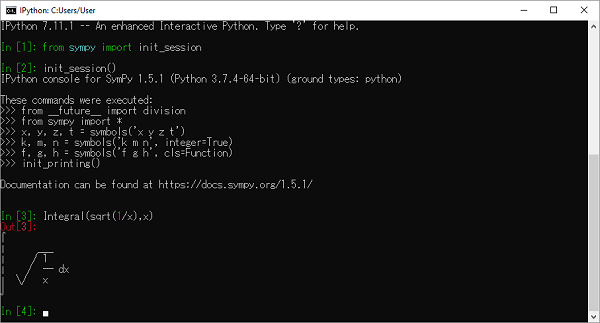To use ASCII printer use pprint() function with use_unicode property set to False

>>> pprint(Integral(sqrt(1/x),x),use_unicode=False)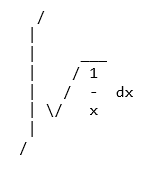The Unicode pretty printer is also accessed from pprint() and pretty(). If the terminal supports Unicode, it is used automatically. If pprint() is not able to detect that the terminal supports unicode, you can pass use_unicode=True to force it to use Unicode.

To get the LATEX form of an expression, use latex() function.

>>> print(latex(Integral(sqrt(1/x),x)))


You can also use mathml printer. for that purpose, import print_mathml function. A string version is obtained by mathml() function.

>>> from sympy.printing.mathml import print_mathml
>>> print_mathml(Integral(sqrt(1/x),x))

>>>mathml(Integral(sqrt(1/x),x))


## Acoth¶

class sympy.functions.elementary.hyperbolic.acoth

The inverse hyperbolic cotangent function.

• acoth(x) -> Returns the inverse hyperbolic cotangent of x
inverse(argindex=1)

Returns the inverse of this function.

## Arg¶

Returns the argument (in radians) of a complex number. For a real
number, the argument is always 0.




class sympy.functions.elementary.complexes.arg

Returns the argument (in radians) of a complex number

## Powersimp

This function reduces given expression by combining powers with similar bases and exponents.

>>> expr=x**y*x**z*y**z
>>> expr


The above code snippet gives an output equivalent to the below expression −

$x^y x^z y^z$

>>> powsimp(expr)


The above code snippet gives an output equivalent to the below expression −

You can make powsimp() only combine bases or only combine exponents by changing combine=’base’ or combine=’exp’. By default, combine=’all’, which does both.If force is True then bases will be combined without checking for assumptions.

>>> powsimp(expr, combine='base', force=True)


The above code snippet gives an output equivalent to the below expression −

## Floor¶

class sympy.functions.elementary.integers.floor

Floor is a univariate function which returns the largest integer
value not greater than its argument. However this implementation
generalizes floor to complex numbers.

pp. 87 or visit http://mathworld.wolfram.com/FloorFunction.html.





## Max¶

Returns the maximum of two (comparable) expressions

It is named and not to avoid conflicts with the built-in function .

class sympy.functions.elementary.miscellaneous.Max

Return, if possible, the maximum value of the list.

When number of arguments is equal one, then
return this argument.

When number of arguments is equal two, then
return, if possible, the value from (a, b) that is >= the other.

In common case, when the length of list greater than 2, the task
is more complicated. Return only the arguments, which are greater
than others, if it is possible to determine directional relation.

If is not possible to determine such a relation, return a partially
evaluated result.

Assumptions are used to make the decision too.

Also, only comparable arguments are permitted.

find minimum values













Max(x, y, z)





The source values are sequentially allocated by the isolated subsets
in which supremums are searched and result as Max arguments.

If the resulted supremum is single, then it is returned.

The isolated subsets are the sets of values which are only the comparable
with each other in the current set. E.g. natural numbers are comparable with
each other, but not comparable with the symbol. Another example: the
symbol with negative assumption is comparable with a natural number.

Also there are “least” elements, which are comparable with all others,
and have a zero property (maximum or minimum for all elements). E.g. .
In case of it the allocation operation is terminated and only this value is
returned.

Assumption:
• if A > B > C then A > C
• if A==B then B can be removed
Дополнительно:  Скачать программу freedom на андроид с root правами бесплатно на русском

## Atan¶

class sympy.functions.elementary.trigonometric.atan
• atan(x) will evaluate automatically in the cases
oo, -oo, 0, 1, -1



inverse(argindex=1)

Returns the inverse of this function.

## SymPy — Numbers

The core module in SymPy package contains Number class which represents atomic numbers. This class has two subclasses: Float and Rational class. Rational class is further extended by Integer class.

Float class represents a floating point number of arbitrary precision.

>>> from sympy import Float
>>> Float(6.32)


SymPy can convert an integer or a string to float.

>>> Float(10)

Float('1.33E5')# scientific notation


While converting to float, it is also possible to specify number of digits for precision as given below −

>>> Float(1.33333,2)


A representation of a number (p/q) is represented as object of Rational class with q being a non-zero number.

>>> Rational(3/4)


If a floating point number is passed to Rational() constructor, it returns underlying value of its binary representation

>>> Rational(0.2)


For simpler representation, specify denominator limitation.

>>> Rational(0.2).limit_denominator(100)


When a string is passed to Rational() constructor, a rational number of arbitrary precision is returned.

>>> Rational("3.65")


Rational object can also be obtained if two number arguments are passed. Numerator and denominator parts are available as properties.

>>> a=Rational(3,5)
>>> print (a)
>>> print ("numerator:{}, denominator:{}".format(a.p, a.q))

>>> a


Integer class in SymPy represents an integer number of any size. The constructor can accept a Float or Rational number, but the fractional part is discarded

>>> Integer(10)

>>> Integer(3.4)

>>> Integer(2/7)


SymPy has a RealNumber class that acts as alias for Float. SymPy also defines Zero and One as singleton classes accessible with S.Zero and S.One respectively as shown below −

>>> S.Zero

>>> S.One


Other predefined Singleton number objects are Half, NaN, Infinity and ImaginaryUnit

>>> from sympy import S
>>> print (S.Half)

>>> print (S.NaN)


Infinity is available as oo symbol object or S.Infinity

>>> from sympy import oo
>>> oo

>>> S.Infinity


ImaginaryUnit number can be imported as I symbol or accessed as S.ImaginaryUnit and represents square root of -1

>>> from sympy import I
>>> I

>>> S.ImaginaryUnit

>>> from sympy import sqrt
>>> i=sqrt(-1)
>>> i*i


## Point

>>> from sympy.geometry import Point
>>> from sympy import *
>>> x=Point(0,0)
>>> y=Point(2,2)
>>> z=Point(4,4)
>>> Point.is_collinear(x,y,z)

>>> a=Point(2,3)
>>> Point.is_collinear(x,y,a)


The distance() method of Point class calculates distance between two points

>>> x.distance(y)


The distance may also be represented in terms of symbols.

## Sqrt¶

Returns the square root of an expression. It is equivalent to raise to .




class sympy.functions.elementary.miscellaneous.sqrt

The square root function

sqrt(x) -> Returns the principal square root of x.





Note that sqrt(x**2) does not simplify to x.

This is because the two are not equal to each other in general.
For example, consider x == -1:





This is because sqrt computes the principal square root, so the square may
put the argument in a different branch. This identity does hold if x is
positive:





You can force this simplification by using the powdenest() function with
the force option set to True:





To get both branches of the square root you can use the RootOf function:







## Im¶

Returns the imaginary part of an expression.





## Sinh¶

class sympy.functions.elementary.hyperbolic.sinh
• sinh(x) -> Returns the hyperbolic sine of x

, ,

as_real_imag(deep=True, **hints)

Returns this function as a complex coordinate.

fdiff(argindex=1)

Returns the first derivative of this function.

inverse(argindex=1)

Returns the inverse of this function.

static taylor_term(*args, **kw_args)

Returns the next term in the Taylor series expansion.

## Conjugate¶

Returns the complex conjugate
of an argument. In mathematics, the complex conjugate of a complex number is given
by changing the sign of the imaginary part. Thus, the conjugate of the complex number

$$a + ib$$

(where a and b are real numbers) is

$$a — ib$$




class sympy.functions.elementary.complexes.conjugate

Changes the sign of the imaginary part of a complex number.







## Tanh¶

class sympy.functions.elementary.hyperbolic.tanh
• tanh(x) -> Returns the hyperbolic tangent of x

, ,

inverse(argindex=1)

Returns the inverse of this function.

## Combsimp

Combinatorial expressions involving factorial an binomials can be simplified by using combsimp() function. SymPy provides a factorial() function

>>> expr=factorial(x)/factorial(x - 3)
>>> expr


The above code snippet gives an output equivalent to the below expression −

>>> combsimp(expr)


The above code snippet gives an output equivalent to the below expression −

The binomial(x, y) is the number of ways to choose y items from a set of x distinct items. It is also often written as xCy.

>>> binomial(x,y)


The above code snippet gives an output equivalent to the below expression −

>>> combsimp(binomial(x+1, y+1)/binomial(x, y))


The above code snippet gives an output equivalent to the below expression −

## Atan2¶

class sympy.functions.elementary.trigonometric.atan2

Attention: Note the role reversal of both arguments. The -coordinate
is the first argument and the -coordinate the second.

, , , , , , , ,









-y/(x**2 + y**2)


x/(x**2 + y**2)



-I*log((x + I*y)/sqrt(x**2 + y**2))


and in terms of :



2*atan(y/(x + sqrt(x**2 + y**2)))


but note that this form is undefined on the negative real axis.

## SymPy — evalf() function

>>> from sympy.abc import r
>>> expr=pi*r**2
>>> expr


The above code snippet gives an output equivalent to the below expression −

To evaluate above expression using evalf() function by substituting r with 5

>>> expr.evalf(subs={r:5})

>>> expr=a/b
>>> expr.evalf(20, subs={a:100, b:3})


## SymPy — Matrices

In Mathematics, a matrix is a two dimensional array of numbers, symbols or expressions. Theory of matrix manipulation deals with performing arithmetic operations on matrix objects, subject to certain rules.

Linear transformation is one of the important applications of matrices. Many scientific fields, specially related to Physics use matrix related applications.

SymPy package has matrices module that deals with matrix handling. It includes Matrix class whose object represents a matrix.

Note: If you want to execute all the snippets in this chapter individually, you need to import the matrix module as shown below −

>>> from sympy.matrices import Matrix

>>> from sympy.matrices import Matrix
>>> m=Matrix([[1,2,3],[2,3,1]])
>>> m
$\displaystyle \left[\begin{matrix}1 & 2 & 3\\2 & 3 & 1\end{matrix}\right]$


Matrix is created from appropriately sized List objects. You can also obtain a matrix by distributing list items in specified number of rows and columns.

>>> M=Matrix(2,3,[10,40,30,2,6,9])
>>> M
$\displaystyle \left[\begin{matrix}10 & 40 & 30\\2 & 6 & 9\end{matrix}\right]$


Matrix is a mutable object. The matrices module also provides ImmutableMatrix class for obtaining immutable matrix.

## Combinatorial functions

Combinatorics is a field of mathematics concerned with problems of selection, arrangement, and operation within a finite or discrete system.

The factorial is very important in combinatorics where it gives the number of ways in which n objects can be permuted. It is symbolically represented as ð‘¥! This function is implementation of factorial function over nonnegative integers, factorial of a negative integer is complex infinity.

>>> x=Symbol('x')
>>> factorial(x)


The output for the above code snippet is given below −

>>> factorial(5)


The output for the above code snippet is given below −

>>> factorial(-1)


The output for the above code snippet is given below −

## Nand Function

This function performs Logical NAND operation. It evaluates its arguments and returns True if any of them are False, and False if they are all True.

>>> from sympy import *
>>> from sympy.logic.boolalg import Nand
>>> a,b,c=symbols('a b c')
>>> a,b,c=(True, False, True)
>>> Nand(a,b,c), Nand(a,c)


## SymPy — Function class

Sympy package has Function class, which is defined in sympy.core.function module. It is a base class for all applied mathematical functions, as also a constructor for undefined function classes.

• Functions for complex number
• Trigonometric functions
• Functions for integer number
• Combinatorial functions
• Other miscellaneous functions

## Tan¶

class sympy.functions.elementary.trigonometric.tan
• tan(x) will evaluate automatically in the case x is a
multiple of pi.


2*x*(tan(x**2)**2 + 1)


inverse(argindex=1)

Returns the inverse of this function.

## Cancel

The cancel() function will take any rational function and put it into the standard canonical form, p/q, where p and q are expanded polynomials with no common factors. The leading coefficients of p and q do not have denominators i.e., they are integers.

>>> expr1=x**2+2*x+1
>>> expr2=x+1
>>> cancel(expr1/expr2)


The above code snippet gives an output equivalent to the below expression −

>>> expr = 1/x + (3*x/2 - 2)/(x - 4)
>>> expr


The above code snippet gives an output equivalent to the below expression −

>>> cancel(expr)


The above code snippet gives an output equivalent to the below expression −

>>> expr=1/sin(x)**2
>>> expr1=sin(x)
>>> cancel(expr1*expr)


The above code snippet gives an output equivalent to the below expression −

## LambertW¶

class sympy.functions.elementary.exponential.LambertW

Lambert W function, defined as the inverse function of
x*exp(x). This function represents the principal branch
of this inverse function, which like the natural logarithm
is multivalued.

fdiff(argindex=1)

Return the first derivative of this function.

## Ellipse

An elliptical geometry entity is constructed by passing a Point object corresponding to center and two numbers each for horizontal and vertical radius.

>>> from sympy.geometry import Ellipse, Line
>>> e=Ellipse(Point(0,0),8,3)
>>> e.area


The vradius can be indirectly provided by using eccentricity parameter.

>>> e1=Ellipse(Point(2,2), hradius=5, eccentricity=Rational(3,4))


The apoapsis of the ellipse is the greatest distance between the focus and the contour.

>>> e1.apoapsis

>>> e1.circumference


The equation method of ellipse returns equation of ellipse.

>>> e1.equation(x,y)


## Non-linear equation

For this purpose, we use nonlinsolve() function. Equations for this example −

>>> a,b=symbols('a b')
>>> nonlinsolve([a**2 + a, a - b], [a, b])


## Linear equation

We have to use linsolve() function to solve linear equations.

>>> from sympy import *
>>> x,y=symbols('x y')
>>> linsolve([Eq(x-y,4),Eq( x + y ,1) ], (x, y))


The linsolve() function can also solve linear equations expressed in matrix form.

>>> a,b=symbols('a b')
>>> a=Matrix([[1,-1],[1,1]])
>>> b=Matrix([4,1])
>>> linsolve([a,b], (x,y))


## Re¶

Return the real part of an expression.




class sympy.functions.elementary.complexes.re

Returns real part of expression. This function performs only
elementary analysis and so it will fail to decompose properly
more complicated expressions. If completely simplified result
is needed then use Basic.as_real_imag() or perform complex
expansion on instance of this function.







as_real_imag(deep=True, **hints)

Returns the real number with a zero complex part.

## SymPy — Symbolic Computation

Symbolic computation refers to development of algorithms for manipulating mathematical expressions and other mathematical objects. Symbolic computation integrates mathematics with computer science to solve mathematical expressions using mathematical symbols. A Computer Algebra System (CAS) such as SymPy evaluates algebraic expressions exactly (not approximately) using the same symbols that are used in traditional manual method. For example, we calculate square root of a number using Python’s math module as given below −

>>> import math
>>> print (math.sqrt(25), math.sqrt(7))


As you can see, square root of 7 is calculated approximately. But in SymPy square roots of numbers that are not perfect squares are left unevaluated by default as given below −

>>> import sympy
>>> print (sympy.sqrt(7))


It is possible to simplify and show result of expression symbolically with the code snippet below −

>>> import math
>>> print (math.sqrt(12))


You need to use the below code snippet to execute the same using sympy −

##sympy output
>>> print (sympy.sqrt(12))


SymPy code, when run in Jupyter notebook, makes use of MathJax library to render mathematical symbols in LatEx form. It is shown in the below code snippet −

>>> from sympy import *
>>> x=Symbol ('x')
>>> expr = integrate(x**x, x)
>>> expr

Integral(x**x, x)


Which is equivalent to

>>> from sympy import *
>>> x=7
>>> sqrt(x)


A symbolic computation system such as SymPy does all sorts of computations (such as derivatives, integrals, and limits, solve equations, work with matrices) symbolically. SymPy package has different modules that support plotting, printing (like LATEX), physics, statistics, combinatorics, number theory, geometry, logic, etc.

## SymPy — Installation

pip install sympy

>>> import sympy
>>> sympy.__version__


And you get the below output as the current version of sympy −

Source code of SymPy package is available at https://github.com/sympy/sympy.

This method available in Quaternion class performs addition of two Quaternion objects.

>>> q1=Quaternion(1,2,3,4)
>>> q2=Quaternion(4,3,2,1)


The above code snippet gives an output equivalent to the below expression −

$5 + 5i + 5j + 5k$

It is possible to add a number or symbol in a Quaternion object.

>>> q1+2


$3 + 2i + 3j + 4k$

>>> q1+x


$(x + 1) + 2i + 3j + 4k$

## BooleanTrue function

This function is equivalent of True as in core Python. It returns a singleton that can be retrieved by S.true.

>>> from sympy import *
>>> x=sympify(true)
>>> x, S.true


## Pow()

This method returns power of a quaternion object.

>>> q1.pow(2)


$(-8) + 4i + 2j + 4k$

## Matrix Constructors

SymPy provides many special type of matrix classes. For example, Identity matrix, matrix of all zeroes and ones, etc. These classes are named as eye, zeros and ones respectively. Identity matrix is a square matrix with elements falling on diagonal are set to 1, rest of the elements are 0.

from sympy.matrices import eye eye(3)

Matrix([[1, 0, 0], [0, 1, 0], [0, 0, 1]])

$\displaystyle \left[\begin{matrix}1 & 0 & 0\\0 & 1 & 0\\0 & 0 & 1\end{matrix}\right]$


In diag matrix, elements on diagonal are initialized as per arguments provided.

>>> from sympy.matrices import diag
>>> diag(1,2,3)

$\displaystyle \left[\begin{matrix}1 & 0 & 0\\0 & 2 & 0\\0 & 0 & 3\end{matrix}\right]$


All elements in zeros matrix are initialized to 0.

>>> from sympy.matrices import zeros
>>> zeros(2,3)

$\displaystyle \left[\begin{matrix}0 & 0 & 0\\0 & 0 & 0\end{matrix}\right]$


Similarly, ones is matrix with all elements set to 1.

>>> from sympy.matrices import ones
>>> ones(2,3)

$\displaystyle \left[\begin{matrix}1 & 1 & 1\\1 & 1 & 1\end{matrix}\right]$


Оцените статью